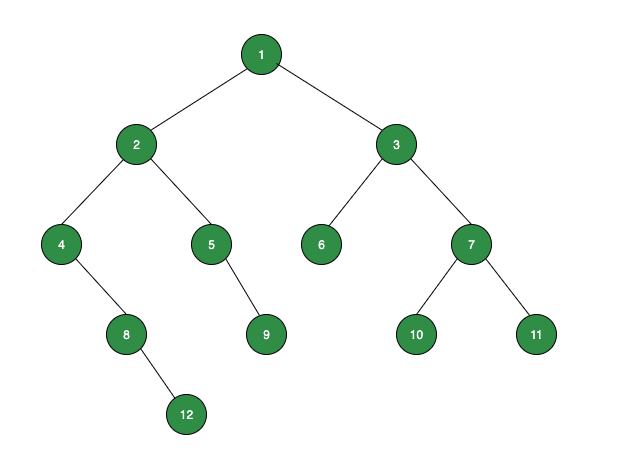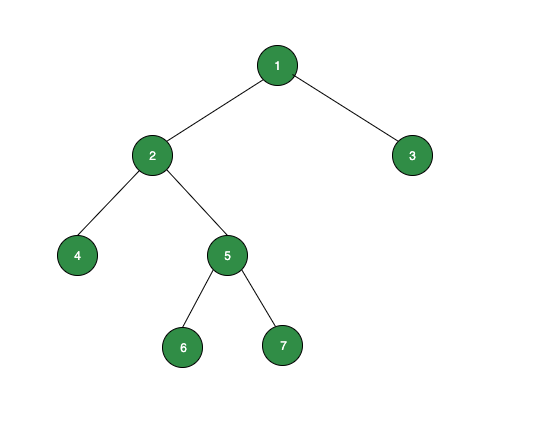# Difference between odd level and even level leaf sum in given Binary Tree

• Difficulty Level : Easy
• Last Updated : 03 Feb, 2022

Given a Binary Tree, the task is to find the difference of the sum of leaf nodes at the odd level and even level of the given tree.

Examples:

Input:Output: -12
Explanation: Following are the operations performed to get the result.
odd_level_sum = 0, even_level_sum = 0
Level 1: No leaf node, so odd_level_sum = 0
Level 2: No leaf node, so even_level_sum = 0
Level 3: One leaf node: 6, so odd_level_sum = 0 + 6 = 6
Level 4: Three leaf nodes: 9, 10, 11, so even_level_sum = 0 + 9 + 10 + 11 = 30
Level 5: One leaf node: 12, so odd_level_sum = 6 + 12 = 18
Therefore, result = odd_level_sum – even_level_sum = 18 – 30 = -12

Input:Output: -12

Approach: The given problem can be solved by using the Level Order Traversal. Follow the steps below to solve the given problem.

• Create a queue q, to store the node. Also, create two variables odd_level_sum and even_level_sum to store the sum of leaf nodes at the odd and even levels of the tree respectively. The other variable level keeps track of the level in the traversal.
• Perform the level order traversal from the root node and store each node in the queue, and also check the current node for the leaf node. If it’s a leaf node then add its value in the odd_level_sum or even_level_sum by checking the level.
• After completing the above steps, print the difference between odd_level_sum and even_level_sum.

Below is the implementation of the above approach:

## C++

 `// C++ program for above approach` `#include ` `using` `namespace` `std;`   `// A tree node structure` `struct` `Node {` `    ``int` `data;` `    ``Node *left, *right;` `};`   `// Utility function to create` `// a new Binary Tree node` `Node* newNode(``int` `data)` `{` `    ``Node* temp = ``new` `Node;` `    ``temp->data = data;` `    ``temp->left = temp->right = NULL;` `    ``return` `temp;` `}`   `// Function to print the difference` `void` `printDifference(Node* root)` `{` `    ``if` `(root == NULL) {` `        ``cout << ``"No nodes present\n"``;` `        ``return``;` `    ``}`   `    ``int` `odd_level_sum = 0,` `        ``even_level_sum = 0,` `        ``level = 1;`   `    ``// queue to hold tree node with level` `    ``queue<``struct` `Node*> q;`   `    ``// Root node is at level 1 so level=1` `    ``q.push(root);`   `    ``// Do level Order Traversal of tree` `    ``while` `(!q.empty()) {` `        ``int` `n = q.size();` `        ``while` `(n--) {` `            ``Node* temp = q.front();` `            ``q.pop();` `            ``if` `(temp->left == NULL` `                ``&& temp->right == NULL) {` `                ``if` `(level & 1) {` `                    ``odd_level_sum += temp->data;` `                ``}` `                ``else` `{` `                    ``even_level_sum += temp->data;` `                ``}` `                ``continue``;` `            ``}` `            ``if` `(temp->left) {` `                ``q.push(temp->left);` `            ``}` `            ``if` `(temp->right) {` `                ``q.push(temp->right);` `            ``}` `        ``}` `        ``level++;` `    ``}` `    ``cout << odd_level_sum - even_level_sum;` `}`   `// Driver Code` `int` `main()` `{` `    ``Node* root = newNode(1);` `    ``root->left = newNode(2);` `    ``root->right = newNode(3);` `    ``root->left->left = newNode(4);` `    ``root->left->right = newNode(5);` `    ``root->right->left = newNode(6);` `    ``root->right->right = newNode(7);` `    ``root->left->left->right = newNode(8);` `    ``root->left->right->right = newNode(9);` `    ``root->right->right->left = newNode(10);` `    ``root->right->right->right = newNode(11);` `    ``root->left->left->right->right = newNode(12);`   `    ``printDifference(root);`   `    ``return` `0;` `}`

## Java

 `// Java program for above approach` `import` `java.util.LinkedList;` `import` `java.util.Queue;`   `class` `GFG {`   `  ``// A tree node structure` `  ``static` `class` `Node {` `    ``int` `data;` `    ``Node left;` `    ``Node right;`   `    ``public` `Node(``int` `data) {` `      ``this``.data = data;` `      ``this``.left = ``null``;` `      ``this``.right = ``null``;` `    ``}` `  ``};`   `  ``// Utility function to create` `  ``// a new Binary Tree node` `  ``static` `Node Node(``int` `data) {` `    ``Node temp = ``new` `Node(``0``);` `    ``temp.data = data;` `    ``temp.left = temp.right = ``null``;` `    ``return` `temp;` `  ``}`   `  ``// Function to print the difference` `  ``static` `void` `printDifference(Node root) {` `    ``if` `(root == ``null``) {` `      ``System.out.println(``"No nodes present"``);` `      ``return``;` `    ``}`   `    ``int` `odd_level_sum = ``0``,` `    ``even_level_sum = ``0``,` `    ``level = ``1``;`   `    ``// queue to hold tree node with level` `    ``Queue q = ``new` `LinkedList();`   `    ``// Root node is at level 1 so level=1` `    ``q.add(root);`   `    ``// Do level Order Traversal of tree` `    ``while` `(!q.isEmpty()) {` `      ``int` `n = q.size();` `      ``while` `(n-- > ``0``) {` `        ``Node temp = q.peek();` `        ``q.remove();` `        ``if` `(temp.left == ``null` `            ``&& temp.right == ``null``) {` `          ``if` `((level & ``1``) > ``0``) {` `            ``odd_level_sum += temp.data;` `          ``} ``else` `{` `            ``even_level_sum += temp.data;` `          ``}` `          ``continue``;` `        ``}` `        ``if` `(temp.left != ``null``) {` `          ``q.add(temp.left);` `        ``}` `        ``if` `(temp.right != ``null``) {` `          ``q.add(temp.right);` `        ``}` `      ``}` `      ``level++;` `    ``}` `    ``System.out.println(odd_level_sum - even_level_sum);` `  ``}`   `  ``// Driver Code` `  ``public` `static` `void` `main(String args[]) {` `    ``Node root = ``new` `Node(``1``);` `    ``root.left = ``new` `Node(``2``);` `    ``root.right = ``new` `Node(``3``);` `    ``root.left.left = ``new` `Node(``4``);` `    ``root.left.right = ``new` `Node(``5``);` `    ``root.right.left = ``new` `Node(``6``);` `    ``root.right.right = ``new` `Node(``7``);` `    ``root.left.left.right = ``new` `Node(``8``);` `    ``root.left.right.right = ``new` `Node(``9``);` `    ``root.right.right.left = ``new` `Node(``10``);` `    ``root.right.right.right = ``new` `Node(``11``);` `    ``root.left.left.right.right = ``new` `Node(``12``);`   `    ``printDifference(root);`   `  ``}` `}`   `// This code is contributed by saurabh_jaiswal.`

Output

`-12`

Time Complexity: O(N)
Space Complexity: O(N)

My Personal Notes arrow_drop_up
Recommended Articles
Page :